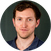# An introduction to statistics for statistical genetics: general statistical conceptsPublished on July 31, 2017   24 min
• Review

#### Other Talks in the Series: Statistical Genetics

Please wait while the transcript is being prepared...
0:00
I'm Dr. Paul O'Reilly, senior lecturer in statistical genetics, performing research at King's College, London. This lecture, "An Introduction to Statistics for Statistical Genetics" is the first talk in the statistical genetics series. This is part one, General Statistical Concepts. If you have a background in statistics, then you may find this section of the talk too basic, in which case you might prefer to skip to part two of the talk, which describes more specific statistical techniques commonly applied in genetic research.
0:30
The point of this talk is to introduce you to some of the key statistical concepts and techniques are important in statistical genetics. My aim here will be to provide you with a basic overview of each of these, along with an illustration of their intuition. Greater detail about the tests and concepts are provided in other lectures in this series and can be found in statistical textbooks or in material online.
0:53
The talk comes in two parts. The first is devoted to providing a brief overview of some general statistical concepts, with the focus on those most relevant to statistical genetics. I'll begin by giving a recap of some of the main summary measures that we use to describe data. I'll then describe some of the fundamental concepts that are central to what statistics is all about, such as models and hypothesis testing. Finally, I'll touch upon one of the biggest controversies in both statistics and science, the Frequentist versus Bayesian debate, as seen through the prism of statistical genetics.
1:28
You should already know these concepts well, but it is worth a quick recap here given their importance in statistical genetics. The mean of a variable, equals the sum of the variable values, divided by the sample size and represents what the variable values would be if they were shared out equally among the individuals. Imagine the height of a group of individuals somehow being shared out equally among them. Their height would then equal the mean height. In genetics, we might be interested in how the mean of a trait such as height, differs between individuals with different forms of a genetic variant.
Hide